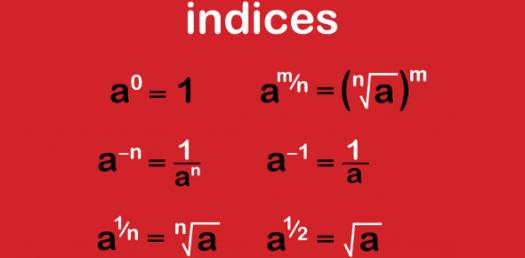# Indices And Standard Form

12 QuestionsSettingsWhat questions can you remember how to do?

Related Topics
• 1.
What is the value of
• A.

6

• B.

9

• C.

8

• D.

5

• 2.
What is
• A.

18

• B.

6

• C.

5

• D.

12

• 3.
What is
• A.

9

• B.

3

• C.

81

• D.

6

• 4.
What is
• A.

30

• B.

100

• C.

1000

• D.

10000

• 5.
Simplify the following:=
• A.
• B.
• C.
• D.
• 6.
Simplify the following:=
• A.
• B.
• C.
• D.
• 7.
Write the following number in standard form:6,000,000,000
• A.
• B.
• C.
• D.
• 8.
What does the following number represent:
• A.

423 000 000

• B.

42 300 000 000

• C.

0.000 000 042 3

• D.

423 000

• 9.
What does the following number represent?
• A.

580 000

• B.

5 800 000

• C.

0.000 58

• D.

0.000 058

• 10.
Evaluate the following:
• A.

5

• B.

12.5

• C.

50

• D.

2.5

• 11.
Evaluate the following:
• A.

-6

• B.

-8

• C.
• D.
• 12.
Evaluate the following:
• A.

18

• B.

9

• C.

81

• D.

3